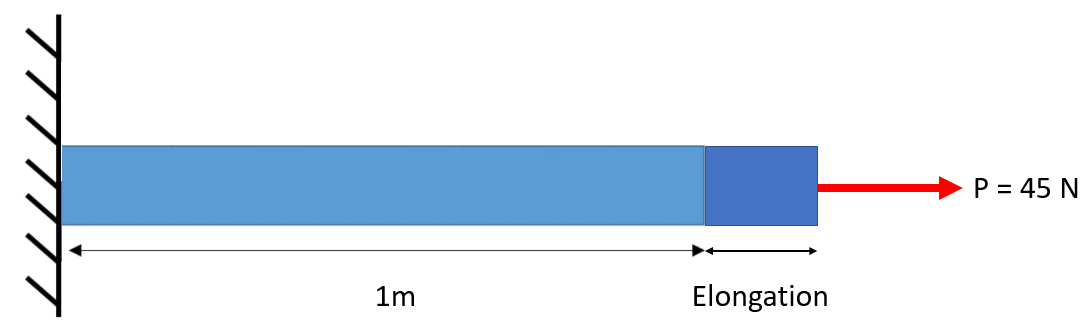## Strain

In the figure below, a 3 cm diameter by 1 m long titanium rod is placed under tension by a 45 N force. If it elongates by 0.05 cm, what is the strain in the rod?Hint
Strain is the change in length per unit length.
Hint 2
$$\varepsilon=\frac{\Delta L}{L_o}$$$where $$\varepsilon$$ is the engineering strain, $$\Delta L$$ is the change in length, and $$L_o$$ is the original length. Strain is the change in length per unit length. $$\varepsilon=\frac{\Delta L}{L_o}$$$
where $$\varepsilon$$ is the engineering strain, $$\Delta L$$ is the change in length, and $$L_o$$ is the original length. Therefore:
$$\varepsilon=\frac{0.05cm}{100m}=0.0005$$\$
0.0005